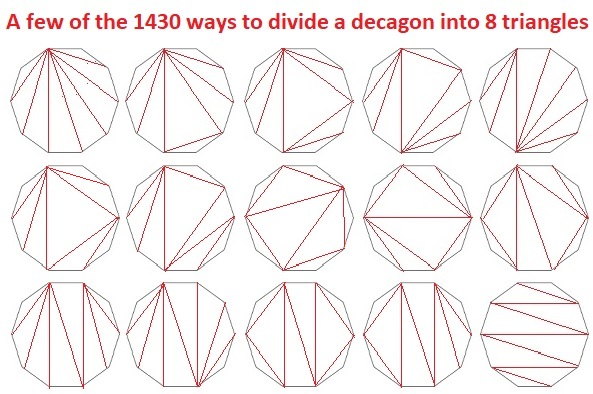# 1430 Is a Catalan Number

1430 is the eighth Catalan number because it is equal to (2⋅8)!÷((8+1)!8!):
10 × 11 × 12 × 13 × 14 × 15 × 16 ÷( 1 × 2 × 3 × 4 × 5 × 6 × 7 × 8 ) = 1430.

For example, the vertices of a decagon can also be the vertices of eight triangles. Those eight triangles can be drawn in 1430 different ways. Here are a few of those ways:Some of the 1430 ways are rotations and/or reflections of the ways illustrated above. Many of the 1430 ways are NOT represented in that graphic at all. It would be mind-boggling to draw all 1430 ways!

Here are some other facts about the number 1430:

• 1430 is a composite number.
• Prime factorization: 1430 = 2 × 5 × 11 × 13.
• 1430 has no exponents greater than 1 in its prime factorization, so √1430 cannot be simplified.
• The exponents in the prime factorization are 1, 1, 1, and 1. Adding one to each exponent and multiplying we get (1 + 1)(1 + 1)(1 + 1)(1 + 1) = 2 × 2 × 2 × 2 = 16. Therefore 1430 has exactly 16 factors.
• The factors of 1430 are outlined with their factor pair partners in the graphic below.1430 is the hypotenuse of FOUR Pythagorean triples:
352-1386-1430 which is 22 times (16-63-65)
550-1320-1430 which is (5-12-13) times 110
726-1232-1430 which is 22 times (33-56-65)
858-1144-1430 which is (3-4-5) times 286